Author: Oscar Cronquist Article last updated on February 25, 2020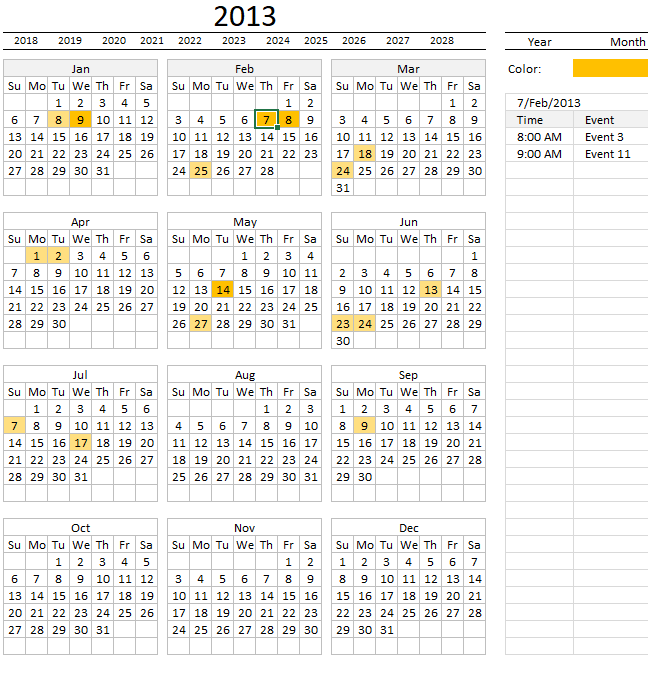The first sheet is named "Calendar" and contains a calendar showing all dates, weekdays and if a date is highlighted it indicates one or more events. The row below the large year number lets you select a year. The calendar changes dates accordingly based on the selected year. See image above.

The Excel Table located in worksheet "Table" lets you specify event date and event text. Days with many "events" have a darker color, days with less "events" have a lighter color and dates without events are not highlighted at all.

The table to the right of the calendar shows all events for a selected day, the image above shows that date February 7th is selected and that date has two events.

Double-clicking on a month takes you to worksheet "Month" with that particular month's events.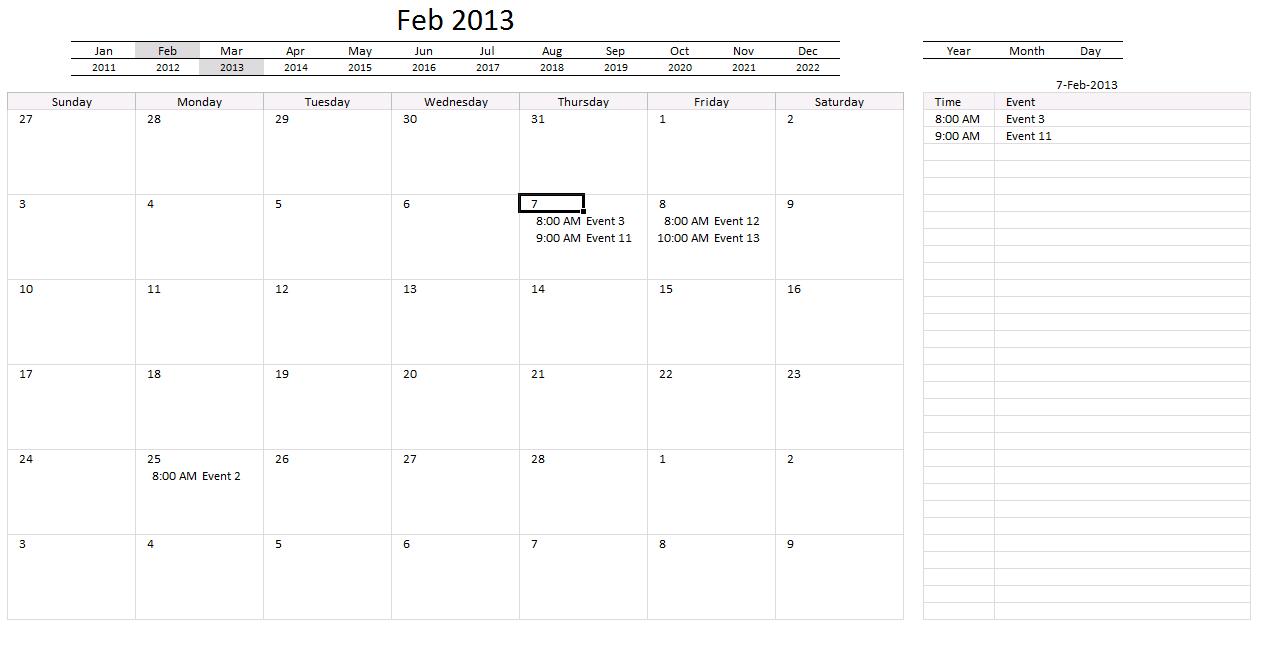The image above shows worksheet "Month", each day shows events. You can also click on a date and the table to the right shows all events for that day.

You can quickly jump between months and years using the top two rows. The first four "events" are shown in each date. Select a date and all events are displayed in the window to the right.

Double-clicking on a date in worksheet "Calendar" takes you to worksheet "Day", see image below.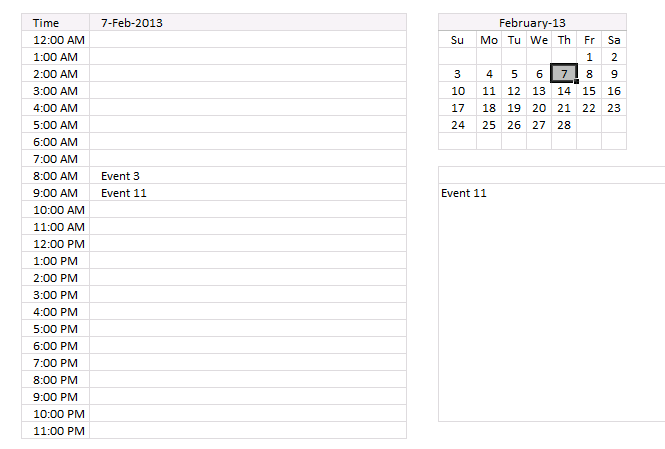Worksheet day shows events based on the date you double-clicked on.

Here you can select a date in the top right calendar, you also select an hour and the corresponding event is shown in the window to the right.

Double click an event and you will be taken to sheet "Table" and the corresponding row, this makes it easy to find and edit a particular event. Likewise, if you double click an empty cell, a new row is created with date and time, see picture below.

Back to top

### Worksheet Table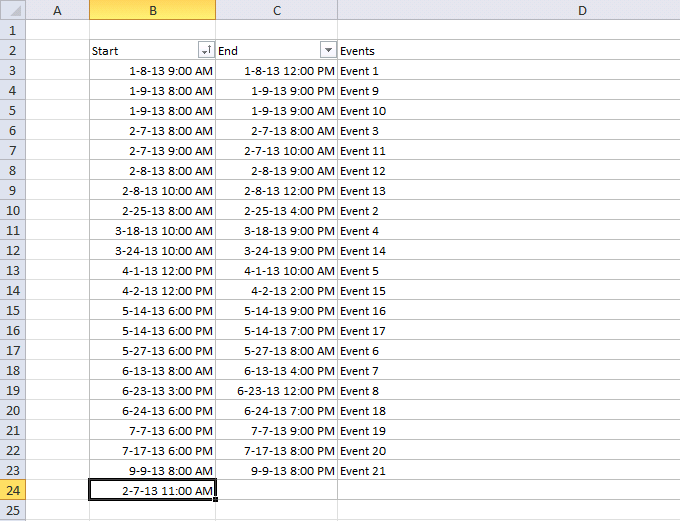This worksheet contains all events, it is also here you add, delete or edit events. The worksheet contains an Excel Table that grows automatically when you enter new events.

Cell references to an Excel Table are called structured references and look differently than regular cell references.

Regular cell reference:

=A1

Structured reference:

=Table1[Start]

You don't need to adjust these references in formulas which is the greatest advantage of using structured references.

Back to top

### How I built this workbook

#### Formulas and macros in worksheet Calendar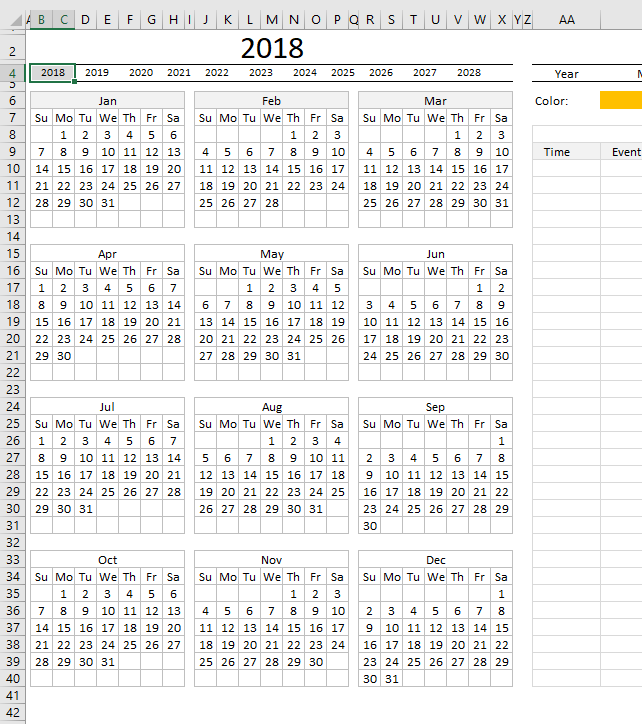The "Calendar" worksheet has a few formulas that make the calendar dynamic meaning the years above the calendar change and dates in the calendar change when you select a year.

Formula in cell B4:

=YEAR(TODAY())-2

Formula in cell D4:

=YEAR(TODAY())-1

The TODAY() function returns the current date, the function is volatile meaning it is recalculated every time any cell in the worksheet is recalculated or calculated.

The YEAR function returns a number representing the current year minus 2. This means that row 4 contains years from two years back up to eight years in the future.

Formula in cell B8:

=DATE(\$K\$2, 1, 1)-WEEKDAY(DATE(\$K\$2, 1, 1), 1)+1

This formula calculates the first date in the first week, note that this date is probably not the first date for the selected year.

The DATE function has three arguments DATE( year, month, day)

Cell \$K\$2 contains the selected year, month is January represented by number 1. 2 is February, 3 is March ... 12 is December.

DATE(\$K\$2, 1, 1)

becomes

DATE(2018, 1, 1)

and returns 43101 which is 1/1/2018, however, it is formatted to only show the day part of the date which is 1.

Excel handles dates differently than you might expect, 1 is 1/1/1900 and 1/1/2000 is 36526, there are 36526 - 1 equals 36525 meaning there are 36525 days between 1/1/1900 and 1/1/2000.

Try this yourself, type a date in any cell. Select the cell and press CTRL + 1 to show the "Format Cells" dialog box. Click General and check the sample. It shows the number corresponding to the date.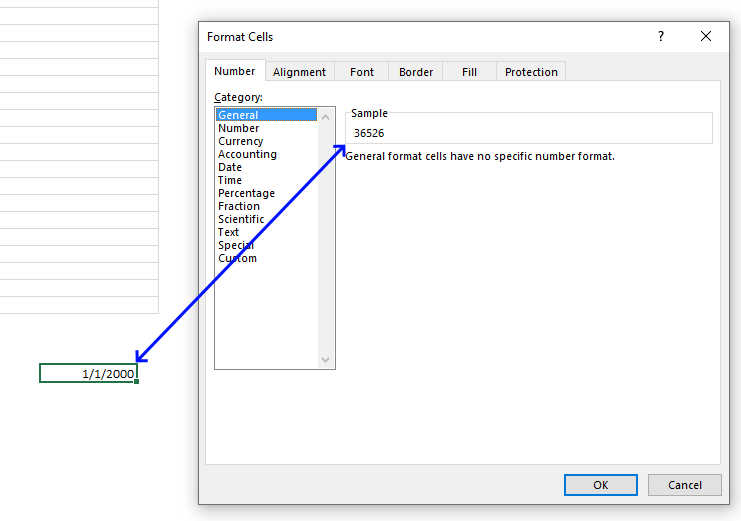```'Event code that is executed if worksheet activated meaning selected
Private Sub Worksheet_Activate()

'Start macro RefreshCal
Call RefreshCal
End Sub
```
```'Event code that is executed before an Excel user doubleclicks a cell
Private Sub Worksheet_BeforeDoubleClick(ByVal Target As Range, Cancel As Boolean)

'Check if cell is in cell range B6:X31
If Not Intersect(Target, Range("B6:X31")) Is Nothing Then

'Check if cell has a formula
If Target.HasFormula Then

'Save value from selected cell to cell C2 worksheet "Day"
Worksheets("Day").Range("C2") = Target.Value

'Go to worksheet "Day"
Worksheets("Day").Activate

'If cell is not in cell range B6:X31 then continue here
Else

'Save value in cell K2 in active worksheet to cell O5 in worksheet "Month"
Worksheets("Month").Range("O5") = ActiveSheet.Range("K2")

'Save value from target cell to cell O4 in worksheet "Month"
Worksheets("Month").Range("O4") = Target.Value

'Go to worksheet "Month"
Worksheets("Month").Activate
End If
End If
End Sub
```
```'This is an event macro that is run when a cell is selected
Private Sub Worksheet_SelectionChange(ByVal Target As Range)

'Check if selected cell is in cell range B8:X31 and the number of selected cells are 1
If Not Intersect(Target, Range("B8:X31")) Is Nothing And Target.Cells.Count = 1 Then

'Save selected cell value to cell AA8
Range("AA8") = Target.Value

'Save selected month to cell O4 in worksheet month
Worksheets("Month").Range("O4") = Evaluate("INDEX(Month!\$C\$4:\$N\$4, MONTH(AA8))")

'Check if selected cell is in cell range B4:W4 and 2 cells are selected
ElseIf Not Intersect(Target, Range("B4:W4")) Is Nothing And Target.Cells.Count = 2 Then

'Save selected cell value to cell K2
Range("K2") = Target.Value

'Save selected cell value to cell O5 in worksheet Month
Worksheets("Month").Range("O5") = Target.Value

'Start macro RefreshCal
Call RefreshCal
Else

'Clear cell AA8
Range("AA8") = ""
End If
End Sub
```

Back to top

#### Formulas and macro in worksheet "Month"```'Event code executed when a cell is selected
Private Sub Worksheet_SelectionChange(ByVal Target As Range)

'Check if selected cell is one of the cells in cell range C4:N4 and that only one cell is selected
If Not Intersect(Target, Range("C4:N4")) Is Nothing And Target.Cells.Count = 1 Then

'Copy selected cell's value to cell O4
Range("O4") = Target.Value

'Check if selected cell is one of the cells in cell range C5:N5 and that only one cell is selected
ElseIf Not Intersect(Target, Range("C5:N5")) Is Nothing And Target.Cells.Count = 1 Then

'Copy selected cell's value to cell O5
Range("O5") = Target.Value

'Copy selected cell's value to cell K2 in worksheet Calendar
Worksheets("Calendar").Range("K2") = Target.Value

'Check if selected cell is one of the cells in cell range B8:O33 and that only one cell is selected and if selected cell's value is a date
ElseIf Not Intersect(Target, Range("B8:O33")) Is Nothing And Target.Cells.Count = 1 And IsDate(Target.Value) Then

'Copy selected cell's value to cell Q6
Range("Q6") = Target.Value

'Check if selected cell is one of the cells in cell range B8:O33
ElseIf Not Intersect(Target, Range("B8:O33")) Is Nothing Then

'Save nothing to cell Q6
Range("Q6") = ""
Else

'Save nothing to cell Q6
Range("Q6") = ""
End If
End Sub
```

Back to top

#### Formulas and macros in worksheet "Day"```'Event macro is executed when a cell is selected
Private Sub Worksheet_SelectionChange(ByVal Target As Range)

'Check if selected cell is one of the cells in cell range E5:K10 and that only one cell is selected
If Not Intersect(Target, Range("E5:K10")) Is Nothing And Target.Cells.Count = 1 Then

'Copy selected cell's value to cell C2
Range("C2") = Target.Value

'Check if selected cell is one of the cells in cell range B3:B26 and that only one cell is selected
ElseIf Not Intersect(Target, Range("B3:B26")) Is Nothing And Target.Cells.Count = 1 Then

'Copy selected cell's value to cell E11
Range("E11") = Target.Value
End If
End Sub
```
```'This event macro is executed before the user doubleclicks on a cell
Private Sub Worksheet_BeforeDoubleClick(ByVal Target As Range, Cancel As Boolean)

'Check if selected cell is one of the cells in cell range C3:C26
If Not Intersect(Target, Range("C3:C26")) Is Nothing Then

'Check if selected cell's value is nothing (empty)
If Target.Value <> "" Then

'Go to worksheet Table
Worksheets("Table").Activate

'Dimension variable and declare data type
Dim Drow As Single

'Find row of the cell that is doubleclicked on based on start date and time, save to variable Drow
Drow = Evaluate("MATCH(" & Round(Target.Offset(0, -1).Value, 4) & ", Round(Table1[Start],4),0)")

'Select that cell in worksheet Table
Worksheets("Table").Range("D" & Drow + 2).Select
Else
Worksheets("Table").Activate
Dim Lrow As Single
Lrow = Worksheets("Table").Range("B" & Rows.Count).End(xlUp).Row
Worksheets("Table").Range("B1").Select
ActiveCell.Offset(Lrow).Select
ActiveCell.Value = Target.Offset(0, -1).Value
End If
End If
End Sub
```

#### Regular macros in the workbook

```'Name macro
Sub RefreshCal()

'Dimension variables and declare data types
Dim CRng As Variant
Dim Dt As Variant
Dim CDt As Variant
Dim Cnt As Integer
Dim r As Long
Dim c As Long
Dim St As Integer

'Disable screen from updating
Application.ScreenUpdating = False

'Save values in cell range B8:X40 to array variable CRng
CRng = Worksheets("Calendar").Range("B8:X40").Value

'The With ... End With statement allows you to write shorter code by referring to an object only once instead of using it with each property.
With Worksheets("Calendar")

'Iterate code between For and Next r based on the number of rows in array variable CRng
For r = 1 To UBound(CRng, 1)

'Iterate code between For and Next c based on the number of columns in array variable CRng
For c = 1 To UBound(CRng, 2)

'
If CRng(r, c) <> "" Then
For CDt = 1 To Range("Table1[Start]").Cells.Count
If CRng(r, c) >= Int(Range("Table1[Start]").Cells(CDt).Value) And CRng(r, c) <= Int(Range("Table1[End]").Cells(CDt).Value) Then Cnt = Cnt + 1 End If Next CDt End If If Cnt > St Then St = Cnt
Cnt = 0
Next c
Next r
End With
Set Rng = Worksheets("Calendar").Range("B8:X40")
'Remove previous formatting
With Rng.Interior
.Pattern = xlNone
.TintAndShade = 0
.PatternTintAndShade = 0
End With
With Worksheets("Calendar")
For Each Dt In Worksheets("Calendar").Range("B6:X38")
For CDt = 1 To Range("Table1[Start]").Cells.Count
If Dt >= Int(Range("Table1[Start]").Cells(CDt).Value) And Dt <= Int(Range("Table1[End]").Cells(CDt).Value) Then Cnt = Cnt + 1 End If Next CDt If Cnt > 0 Then
With Dt.Interior
.Pattern = xlSolid
.PatternColorIndex = xlAutomatic
.Color = Worksheets("Calendar").Range("AB6").Interior.Color ' xlThemeColorAccent4 '
.TintAndShade = 1 - (Cnt / St)
.PatternTintAndShade = 0
End With
'Reset counter a
Cnt = 0
End If
Next Dt
End With
Application.ScreenUpdating = True
End Sub
```

Back to top

### Animated image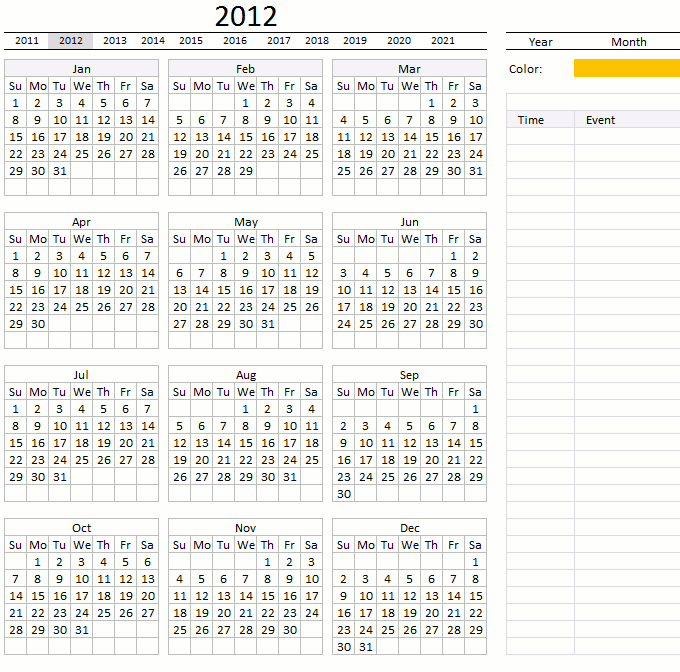Recommended articles

### Download Excel fileYet-another-excel-calendar.xlsm

Back to top